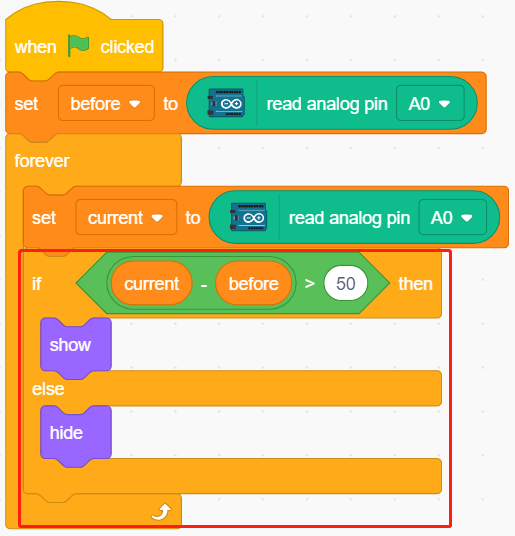# 2.7 Low Temperature Alarm¶

In this project, we will make a low temperature alarm system, when the temperature is below the threshold, the Snowflake sprite will appear on the stage.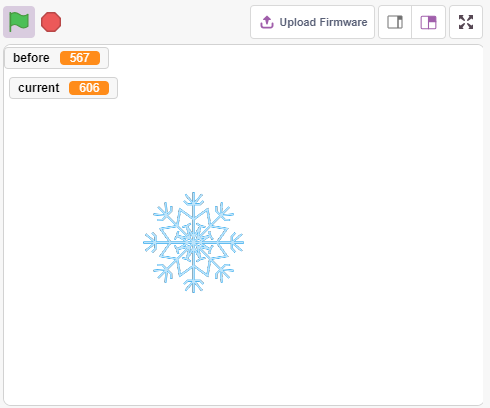## You Will Learn¶

• Thermistor working principle

• Multivariable and Subtractive Operations

## Required Components¶

In this project, we need the following components.

Name

ITEMS IN THIS KIT

3 in 1 Starter Kit

380+

COMPONENT INTRODUCTION

SunFounder R3 Board

Jumper Wires

Resistor

Thermistor

## Build the Circuit¶

A thermistor is a type of resistor whose resistance is strongly dependent on temperature, more so than in standard resistors, and there are two types of resistors, PTC (resistance increases as temperature increases) and PTC (resistance decreases as temperature increases).

Build the circuit according to the following diagram.

One end of the thermistor is connected to GND, the other end is connected to A0, and a 10K resistor is connected in series to 5V.

The NTC thermistor is used here, so when the temperature rises, the resistance of the thermistor decreases, the voltage division of A0 decreases, and the value obtained from A0 decreases, and vice versa increases.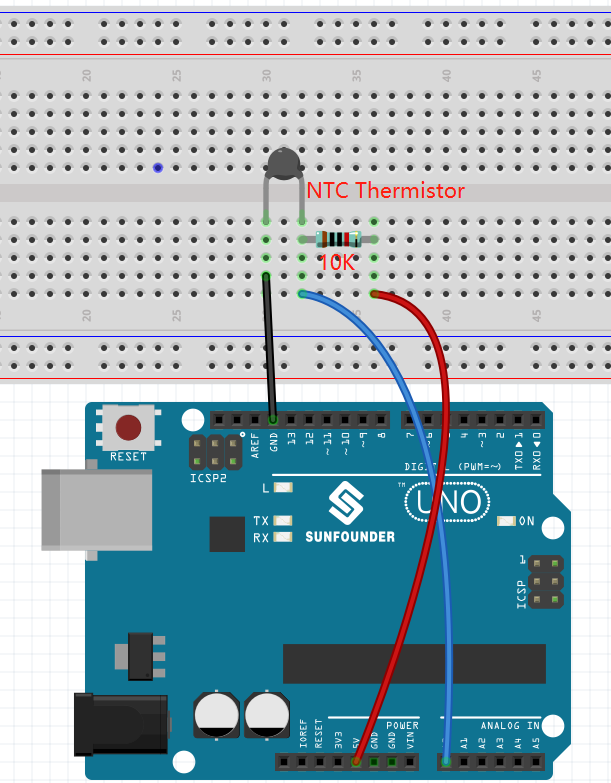## Programming¶

1. Select a sprite

Delete the default sprite, click the Choose a Sprite button in the lower right corner of the sprite area, enter Snowflake in the search box, and then click to add it.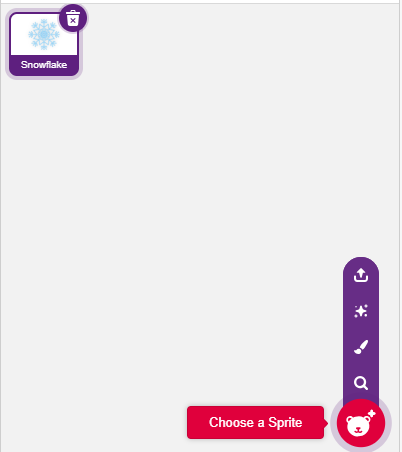2. Create 2 variables

Create two variables, before and current, to store the value of A0 in different cases.3. Read the value of A0

When the green flag is clicked, the value of A0 is read and stored in the variable before.4. Read the value of A0 again

In [forever], read the value of A0 again and store it in the variable current.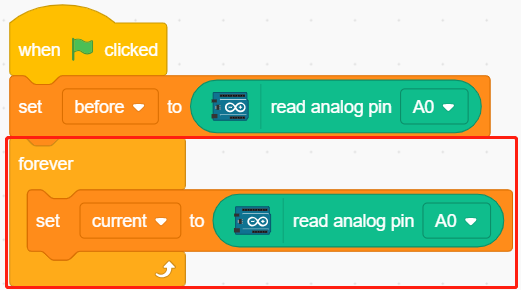5. Determining temperature changes

Using the [if else] block, determine if the current value of A0 is 50 greater than before, which represents a decrease in temperature. At this point let Snowflake sprite show, otherwise hide.

• [-] & [>]: subtraction and comparison operators from Operators platette.